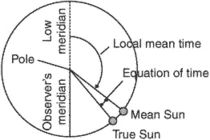# mean sun

Also found in: Dictionary, Thesaurus, Wikipedia.

## mean Sun

An abstract reference point that was introduced to define mean solar time. It has a constant rate of motion and is used in timekeeping in preference to the real Sun whose observed motion is nonuniform. This nonuniformity results primarily from the Earth's elliptical orbit around the Sun, which causes the Earth's orbital speed, and hence the Sun's apparent speed, to vary (see Kepler's laws). Secondly the direction of the Sun's apparent motion is along the ecliptic and is thus inclined to the direction parallel to the celestial equator along which solar time is measured. An additional seasonal variation is therefore introduced when the Sun's motion is measured relative to the equator.

The mean Sun follows a circular orbit around the celestial equator so that it moves eastward at a constant speed and completes one circuit in the same time – one tropical year – as the apparent Sun takes to orbit the ecliptic, if the slight secular acceleration of the Sun is ignored. The mean Sun is defined by an expression for its right ascension, which fixes its position among the stars at every instant.

## Mean Sun

a fictitious point on the celestial sphere that moves uniformly along the celestial equator and crosses the vernal equinox at intervals of a tropical year. The mean sun is used in measuring mean solar time. The concept of the mean sun was introduced in the 18th century to make the measurement of daily time more accurate.

Owing to the eccentricity of the earth’s orbit and the obliquity of the ecliptic, along which the apparent annual motion of the sun occurs, the length of the apparent, or true, solar day—that is, the time interval between successive upper transits of the apparent, or true, sun—varies by as much as 50 seconds in the course of a year. The concept of the mean sun was introduced to eliminate this lack of uniformity. What is known as the equation of time is taken into account when determining mean solar time from observations of the sun. The equation of time is the difference between the right ascensions of the apparent sun and the mean sun. Alternatively, the equation of time can be defined as the difference between the hour angles of the apparent and mean suns.

## mean sun

[′mēn ′sən]
(astronomy)
A fictitious sun conceived to move eastward along the celestial equator at a rate that provides a uniform measure of time equal to the average apparent time; used as a reference for reckoning time, such as mean time or zone time.

## mean sunAn imaginary body which is assumed to travel around the equinoctial at a constant rate, completing one orbit in the same time that the earth takes to complete one orbit around the ecliptic. Because of the elliptical shape of the earth's orbit, the apparent angular velocity of the sun we see is not constant; hence, the days as indicated by the apparent travel of the true or apparent sun are not of uniform length. To make the solar day of uniform length, astronomers have invented the mean sun, a fictitious body imagined to move at a uniform rate along the celestial equator, making a complete circuit from west to east in one year.
References in periodicals archive ?
According to the rule in the Maine Farmers' Almanac, none of the full Moons in 1999 are "blue." Instead, our calculations place four full Moons between the winter solstice of 1999 (determined by the mean Sun) and the Easter Moon of April 2000.
The number of minutes the real Sun lags behind or runs ahead of the mean Sun was named the equation of time.
It is interesting that the midcentury recovery in the number of annual sunny days is much more pronounced than the small rises in mean sun fraction: from minimums of about 25 in the 1920s back to counts exceeding 80 fully sunny days per year by the mid-1960s.
In reality, Local Mean Time is defined as the hour angle of the mean Sun, referred to one's local meridian, plus 12 hours.
SUMMER might mean sun, sea and sand, but it also means our ears have to suffer the onslaught of infectious novelty songs that get playedeverywhere PREPARE FOR DJ DAZ The Woah Song Daz Sampson, one half of Uniting Nations, strikes out on his own with this party anthem.
31.7 Mihre erisim, "Lass uns die Liebste erreichen," Mihr might mean "love" (not "the beloved"), but it can also mean sun and indeed must mean that here, since later in this verse it is contrasted as usual with zerre, "mote" (cf.

Site: Follow: Share:
Open / Close